##### How to Find Array Length in PostgreSQL?

PostgreSQL offers numerous built-in functions to deal with arrays. For instance ARRAY_APPEND(), ARRAY_CAT(), ARRAY_LENGTH(), and so on. All these array functions serve different functionalities. In Postgres, the ARRAY_LENGTH() is one of the most frequently used array functions that find the array's length.

This post will present an in-depth overview of the ARRAY_LENGTH() function with the help of appropriate examples. So, let’s get started!

How to Use the ARRAY_LENGTH() Function in Postgres?

In PostgreSQL, the ARRAY_LENGTH() function accepts two arguments: an array and an integer value that represents the array dimensions to be measured:

`ARRAY_LENGTH(arr, int_val);`

The ARRAY_LENGTH() function will find the array’s length based on the value specified as a second argument. For example, if you set “1” in place of the “int_val” parameter, then the ARRAY_LENGTH() function will find the array length based on the requested dimension, i.e., “1”.

Example 1: How Does ARRAY_LENGTH() Function Work With 1-Dimensional Array in Postgres?

In this example, we will pass a numeric array as the first argument and “1” as the second argument to the ARRAY_LENGTH() function:

`SELECT ARRAY_LENGTH(ARRAY[12, 72, 513, 1, -3, 0], 1);`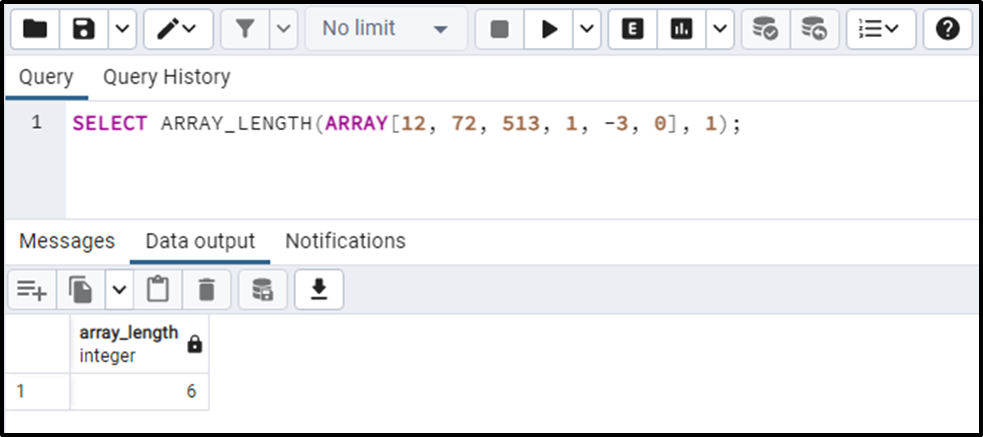The output indicates that the one-dimensional input array has 6 elements in it.

Example 2: How Does ARRAY_LENGTH() Function Work With a 2-Dimensional Array in Postgres?

Let’s learn how to find the length of the 2-D array in Postgres via the ARRAY_LENGTH() function:

`SELECT ARRAY_LENGTH(ARRAY[[12, 72, 513], [1, -3, 0]], 2);`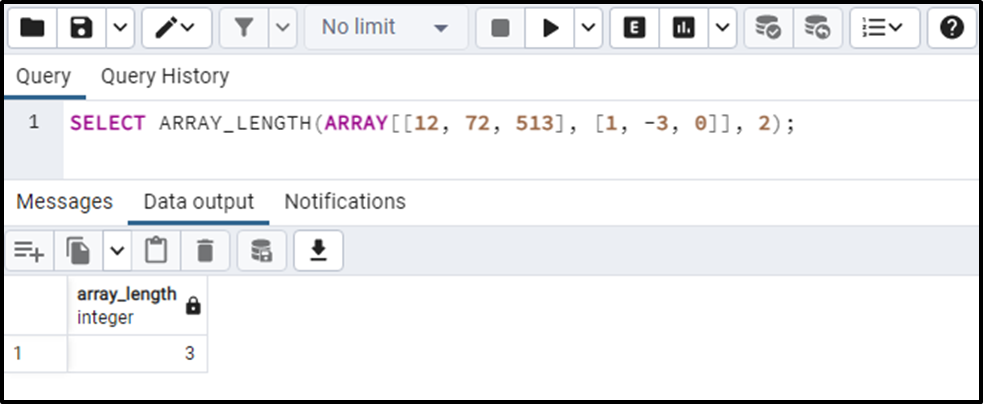The output indicates the length of the two-dimensional input array is “3”.

Similarly, if you have to find the length of a 3-d array, specify “3” as the second parameter to the ARRAY_LENGTH() function, and so on.

Example 3: How Does ARRAY_LENGTH() Function Work on Table’s Data in Postgres?

We have already created a table named “staff_data,” whose data is shown in the following snippet:

`SELECT * FROM staff_data;`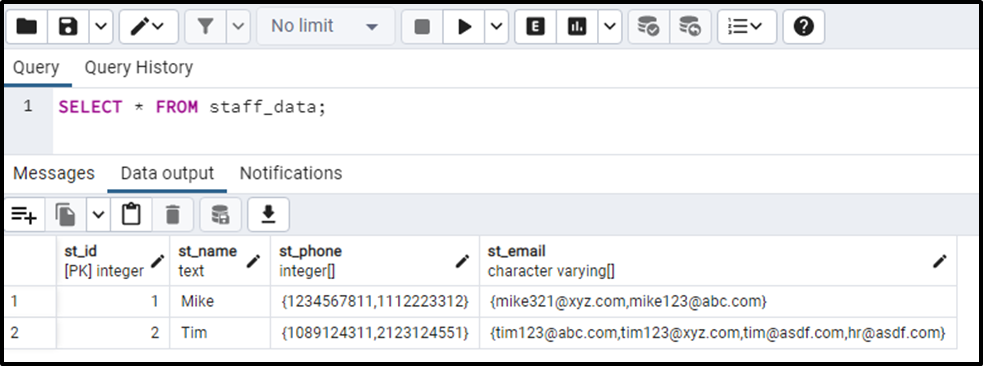Let’s utilize the ARRAY_LENGTH() function to find the length of each record for the “st_email” column:

```SELECT st_email, ARRAY_LENGTH(st_email, 1)
FROM staff_data;```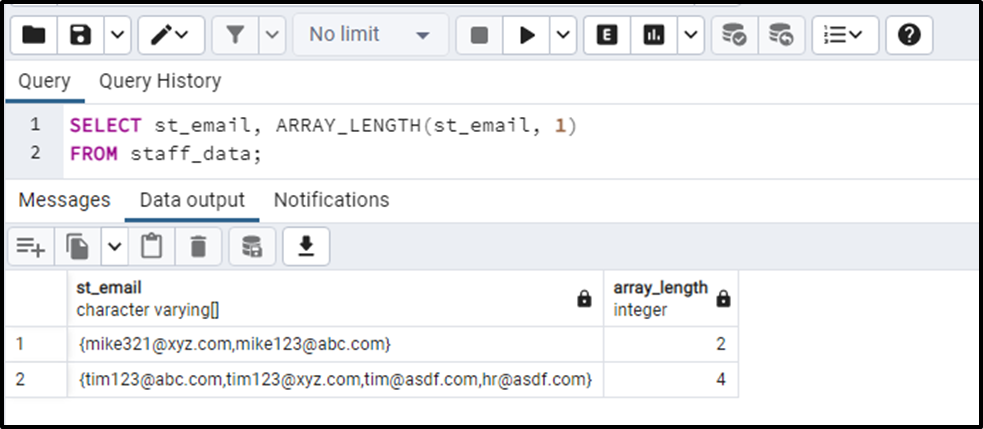The output proves that the ARRAY_LENGTH() function retrieves the appropriate array length for each record.

Example 4: How to Use ARRAY_LENGTH() Function With WHERE Clause in Postgres?

In the previous example, we didn’t specify any condition, so the ARRAY_LENGTH() function finds the length of each array present in the “st_email” column. Use the WHERE clause to find the array length for only a specific record:

```SELECT st_email, ARRAY_LENGTH(st_email, 1)
FROM staff_data
WHERE st_id = 2;```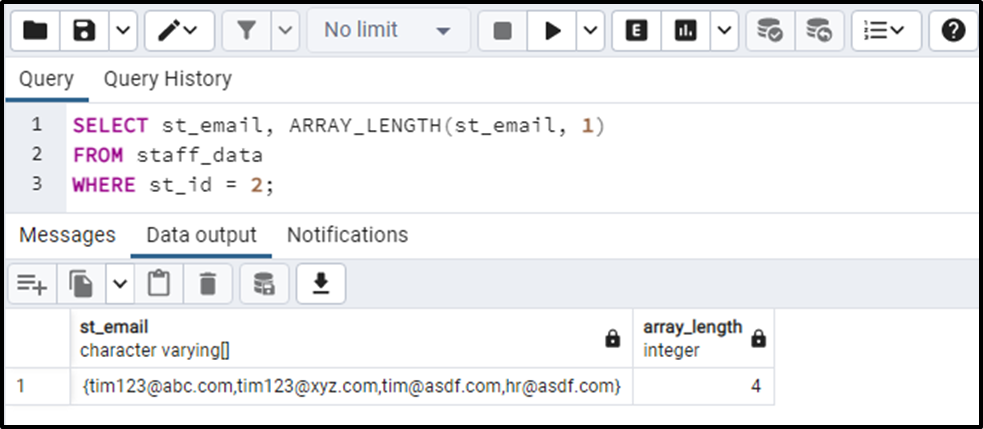This is how you can find the length of only a specific array in Postgres.

Conclusion

In Postgres, the ARRAY_LENGTH() is used to find the array's length. In PostgresQL, the ARRAY_LENGTH() function accepts two arguments: an array and an integer value representing the array dimensions to be measured. The ARRAY_LENGTH() function will find the array’s length based on the requested dimension. This blog post explained how to find the array length in Postgres via the ARRAY_LENGTH() function.